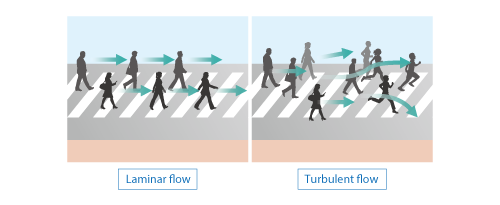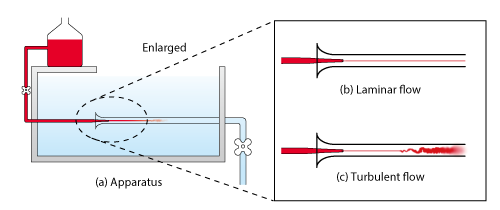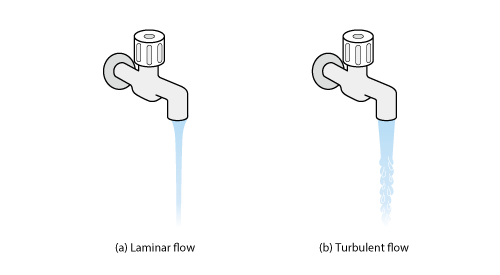# Want to Know More! Basics of Thermo-Fluid Analysis 19: Chapter 3 Flow 3.4.2 Laminar flow and turbulent flow (1)

#### 3.4.2 Laminar flow and turbulent flow (1)

The status of flow is broadly divided into two types. One is flow where a fluid moves regularly in one direction. This kind of flow is called laminar flow . The other is flow where a fluid moves irregularly in many directions. This kind of flow is called turbulent flow . Figure 3.27 shows an image of the two types of flow where people act as flow components.Figure 3.27 Image of laminar flow and turbulent flow

In 1883, a British scientist Osborne Reynolds (1842 - 1912) conducted an experiment and he showed the difference between flows. The experiment is called Reynolds’ experiment . Reynolds used an apparatus as shown in Figure 3.28 (a) to visualize a flow by injecting very thin stream of ink inside a cylinder where water flows. The result illustrated that the stream of ink draws one line when the flow velocity is small as shown in (b). When the flow velocity is large on the other hand, the stream of ink becomes turbulent and diffuses in mid-flow. The former represents laminar flow and the latter turbulent flow.Figure 3.28 Reynolds’ experiment

For an example of phenomena in our daily lives, let us see water from a tap as shown in Figure 3.29. When the amount of water is small, water flows down straight and is laminar flow as shown in (a); however, as the amount of water increases, the water surface starts rippling and the flow changes into turbulent flow as shown in (b).Figure 3.29 Laminar flow and turbulent flow in our daily lives

Based on the experiment described above, Reynolds found a dimensionless number later named Reynolds number that distinguishes between laminar flow and turbulent flow. Reynolds number can be expressed with the following equation using characteristic length L [m], characteristic velocity U [m/s], fluid density ρ [kg/m3], and viscosity coefficient μ [Pa・s]:From the definition that viscosity coefficient divided by density equals kinematic viscosity coefficient ν [m2/s], Reynolds number can also be expressed with the following equation:Reynolds number represents the ratio of inertial force to viscous force. Viscous force is generated by viscosity of a fluid and stabilizes the flow. On the other hand, inertial force is generated by momentum of flow and destabilizes the flow. This means that flow of a small Reynolds number has a large viscous force and is stable, then it is laminar flow. Conversely, when Reynolds number of is large, the flow has a large inertial force and is unstable, then it is turbulent flow.

To image laminar flow, turbulent flow, and each force simply, let us translate a flow to a rotating circle of people holding hands each other. In this example, the strength of held hands corresponds to viscous force and the rotating speed of the circle inertial force. When the strength of held hands is large against the rotaing speed (Reynolds number is small), the circle remains stable (laminar flow); however, when the rotating speed increases (Reynolds number increases), the hands will be released and the circle will be broken (turbulent flow).